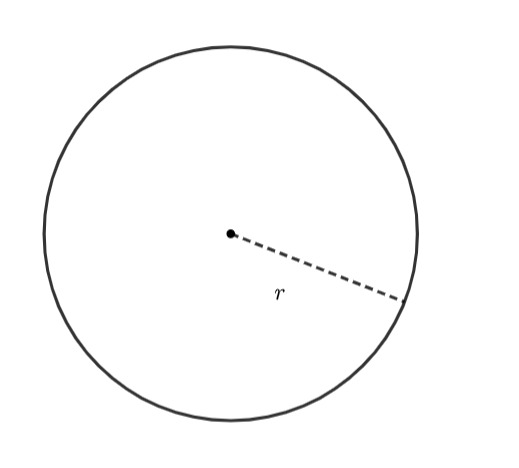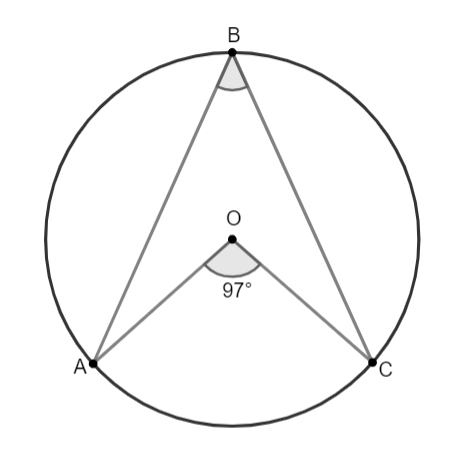Ready to Measure a Circle? Learn Circle Geometry with TutorEye

Did you know that when compared to other shapes that have the same area, a circle would have the smallest perimeter? Learn why and more details about how to calculate various geometrical aspects of a circle.## Some of our best Circle Geometry Tutors

9 Tutors available

## Definition of Circle Geometry:

Circle geometry is a branch of mathematics where we study the properties of circles. Some important aspects of circle geometry are: applying properties of segments intersecting circles, using the relationship between angles in circles, using circles in a coordinate plane.

## Top things to learn under circle geometry from the online tutor

Learn below all the possible concepts about circles you may expect in your geometry exams in simple terms.

• What is a circle?
• Circumference of the Circle
• Circle Theorems
• Area of a Circle
• Tangent
• Arc length of a circle
• The formula for the arc length of a circle
• Chord of a circle
• Area of a sector of a circle
• The angle of a sector of a circle
• Inscribed Angles

## Circle Geometry Frequently Asked Questions:

Question 1: What is a circle in geometry?

In geometry, a circle could be defined as a two-dimensional graphical representation of locus of points that are equal distance from a fixed point called as center.Question 2: What is the center of a circle called in geometry?

The center of a circle is a point that lies in the middle of the circle. It is also referred to as the focus of the circle.

Question 3: What are the 8 circle theorems?

Following are the name of 8 circle theorems.

Angle at the Centre, Angles in a Semicircle, Angles in the Same Segment, Cyclic Quadrilateral, Radius to a Tangent, Tangents from a Point to a Circle, Tangents from a Point to a Circle II, Alternate Segment Theorem.

Question 4: How do you solve a circle in geometry?

To solve problems related to circles in geometry, a good understanding of the circle theorem is necessary.

For example, to solve the unknown angle in the following figure, we could use one of the circle theorems.In the above figure, Angle at the Centre theorem will help to determine the value of the unknown angle.

As per the theorem, the angle at the center is two times greater in measurement than the angle at the circumference.

So, m∠AOC = 2 (m∠ABC)

97° = 2(mABC)

m∠ABC = 48.5°

### Math Tutoring TopicsGet started with a demo session to resolve your queries!Live Circle Geometry Tutoring At Lowest Fee - \$7.49 For 30 Mins/MonthChat With Circle Geometry Tutors Anytime, Anywhere.`Only Pay For Time When You Spend In Circle Geometry ClassroomRefill Anytime With Multiple Of \$7.49 For Online Circle Geometry Lessons

Find A Circle Geometry Tutor Online To Scale Up Your Grades With The Help Of One To One Sessions Online at TutorEye.## Are you looking for fast homework help or regular online Circle Geometry tutoring?Our service is fast, convenient and always offered at any given time!

## Don't Go Far, Look Here!We have the most knowledgeable and experienced online Circle Geometry tutors, who are always available to help you solve a tricky problem, complete a challenging Circle Geometry homework assignment or provide useful test preparation tips.We are arguably the best online tutoring website that offers quality tutoring help at a very attractive costOur service is designed to help both high school and college level students.## Some of Our Key Features!

•## Tutor Verification

TutorEye does a thorough back-check before we employ any Circle Geometry tutor online.

•Out tutors holds teaching license, B.Sc, master's, or Ph.D.

•We offer advanced technological virtual classrooms with complete audio, video and even a whiteboard.

•## No-Technical skill needed

You need no special technical skills to use it.

•## Money back guarantee

Money back guarantee if you are not pleased with us.

•## Works for every student

Our online Circle Geometry tutoring is for everyone who wishes to learn Circle Geometry they can be high school or college level students or anyone with interest in Circle Geometry.

## What students say about us?

98% of our students love us…## We Are Different from Others!